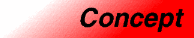MultipleHashedAssociativeContainerCategory: containers Component type: concept

Description

A Multiple Hashed Associative Container is a Hashed Associative Container that is also a MultipleAssociativeContainer. That is, it is a HashedAssociativeContainer with the property that any number of elements in the container may have the same key

Associated types

None, except for those described in the HashedAssociativeContainer and MultipleAssociativeContainer requirements.

Notation

 X A type that is a model of Hashed Associative Container a Object of type X t Object of type X::value_type k Object of type X::key_type p, q Object of type X::iterator n Object of type X::size_type h Object of type X::hasher c Object of type X::key_equal

Valid expressions

In addition to the expressions defined in HashedAssociativeContainer and and MultipleAssociativeContainer, the following expressions must be valid.

Name Expression Type requirements Return type
Range constructor
X(i, j)
X a(i, j);
i and j are InputIterator whose value type is convertible to T . X
Range constructor with bucket count
X(i, j, n)
X a(i, j, n);
i and j are InputIterator whose value type is convertible to T . X
Range constructor with hash function
X(i, j, n, h)
X a(i, j, n, h);
i and j are InputIterator whose value type is convertible to T . X
Range constructor with key equal
X(i, j, n, h, k)
X a(i, j, n, h, k);
i and j are InputIterator whose value type is convertible to T . X

Expression semantics

Name Expression Precondition Semantics Postcondition
Range constructor
X(i, j)
X a(i, j);
[i,j) is a valid range. Creates an associative container that contains all elements in the range [i,j), using hasher() as the hash function and key_equal() as the key equality function. size() is equal to the distance from i to j. The bucket count is an unspecified default value. The hash function is hasher(), and the key equality function is key_equal().
Range constructor with bucket count
X(i, j, n)
X a(i, j, n);
[i,j) is a valid range. Creates an associative container that contains all elements in the range [i,j), using at least n buckets, and using hasher() as the hash function and key_equal() as the key equality function. size() is equal to the distance from i to j. The bucket count is greater than or equal to n. The hash function is hasher(), and the key equality function is key_equal().
Range constructor with hash function
X(i, j, n, h)
X a(i, j, n, h);
[i,j) is a valid range. Creates an associative container that contains all elements in the range [i,j), using at least n buckets, and using h as the hash function and key_equal() as the key equality function. size() is equal to the distance from i to j. The bucket count is greater than or equal to n. The hash function is h, and the key equality function is key_equal().
Range constructor with key equal
X(i, j, n, h, k)
X a(i, j, n, h, k);
[i,j) is a valid range. Creates an associative container that contains all elements in the range [i,j), using at least n buckets, and using h as the hash function and k as the key equality function. size() is equal to the distance from i to j. The bucket count is greater than or equal to n. The hash function is h, and the key equality function is k.

Complexity guarantees

The range constructor, range constructor with bucket count, range constructor with hash function, and range constructor with key equal, are all linear in j - i.